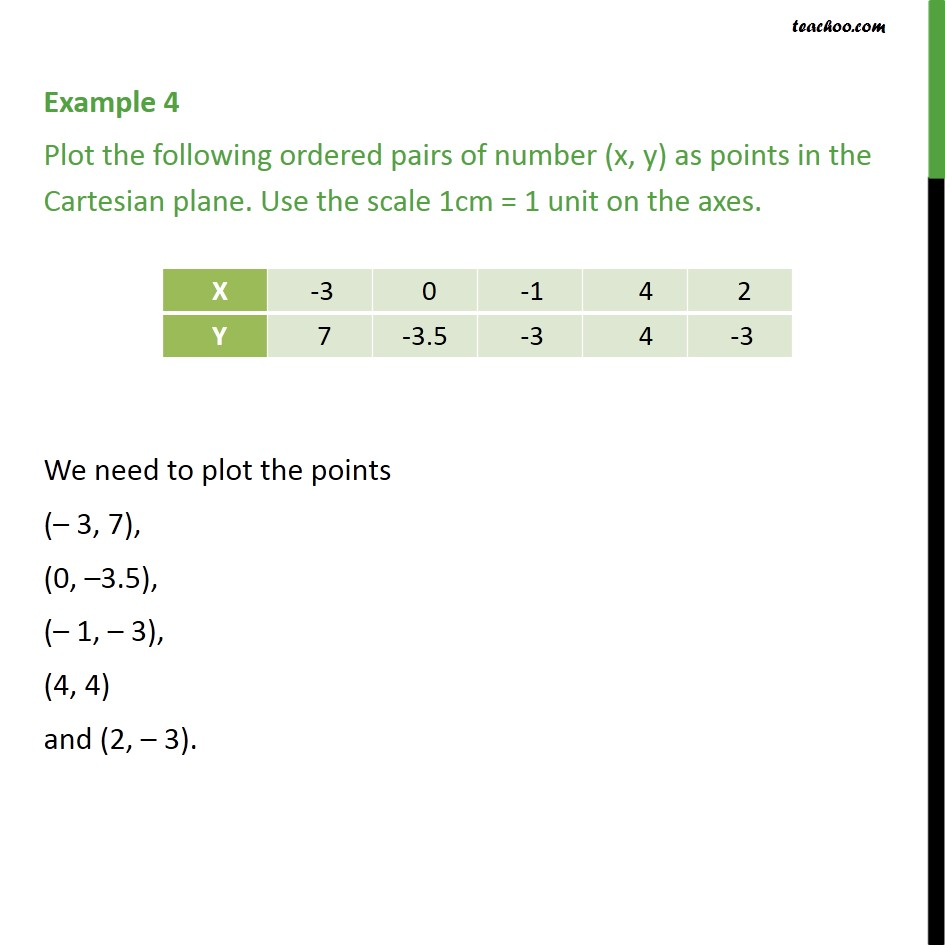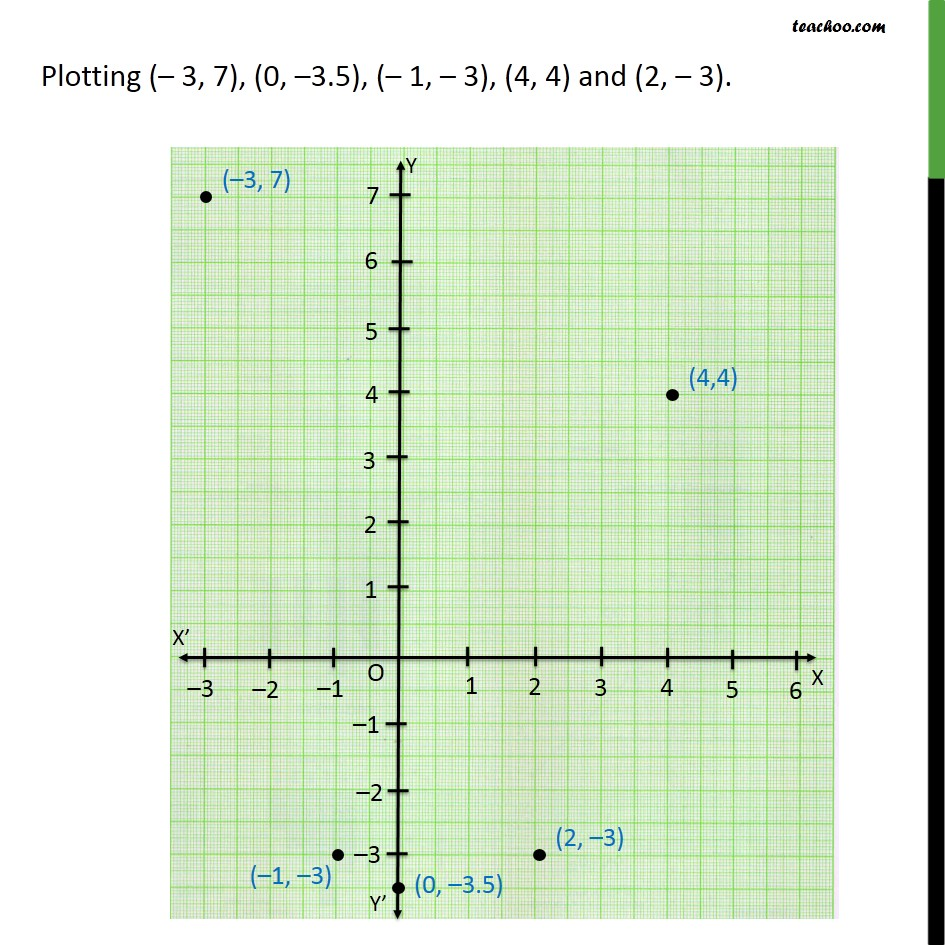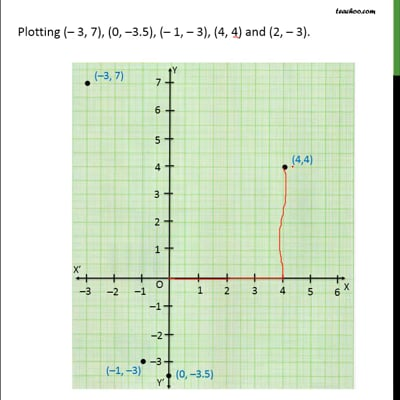Examples

Chapter 3 Class 9 Coordinate Geometry (Term 1)
Serial order wiseThis video is only available for Teachoo black users

### Transcript

Example 4 Plot the following ordered pairs of number (x, y) as points in the Cartesian plane. Use the scale 1cm = 1 unit on the axes. We need to plot the points (– 3, 7), (0, –3.5), (– 1, – 3), (4, 4) and (2, – 3). Plotting (– 3, 7), (0, –3.5), (– 1, – 3), (4, 4) and (2, – 3).